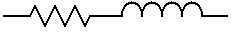# Inductor and Resistor in Series Calculator

This calculator finds the complex impedance (real and imaginary imaginary values) of a capacitor and an inductor in parallel.Frequency: MHz kHz Hz Inductance: mH uH nH Resistance: MΩ kΩ Ω Z =

The complex impedance (Z) (real and imaginary, or resistance and reactance) of an inductor and a resistor in series at a particular frequency can be calculated using the following equation.Where:

f is the Frequency in Hz

L is the Inductance in Henries

R is the Resistance in Ohms

This calculator uses JavaScript and will function in most modern browsers. For more information see About our calculators

This calculator is provided free by Chemandy Electronics in order to promote the FLEXI-BOX

Return to Calculator Index